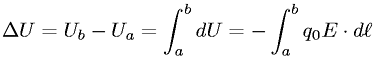Equations > Physics > Electricity and Magnetism > Change in electrostatic potential energy for a point charge

### Change in electrostatic potential energy for a point chargeLatex Code:

MathML Code:

 $\Delta U={U}_{b}-{U}_{a}={\int }_{a}^{b}\mathrm{dU}=-{\int }_{a}^{b}{q}_{0}E·d\ell$

MathType 5.0: